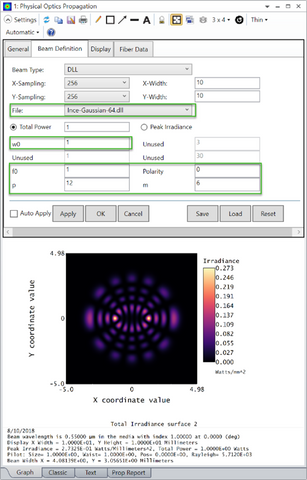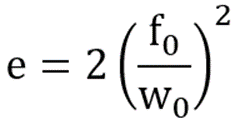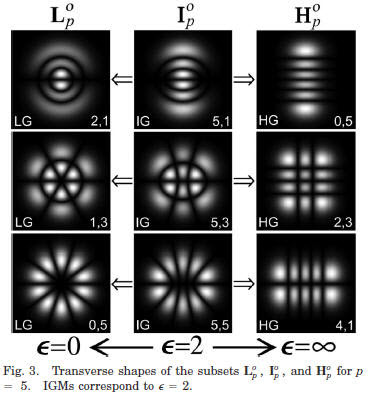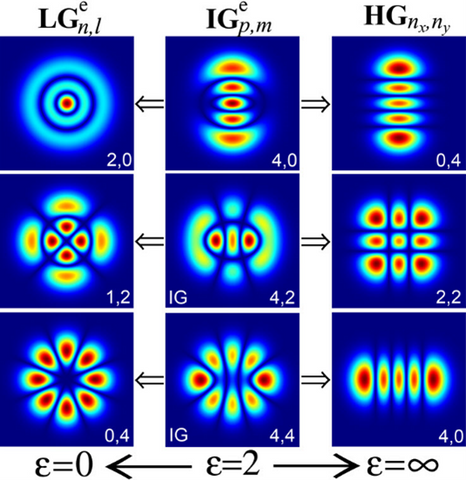## Modeling high order laser beams in OpticStudio

Category: Product NewsIn general, the output of a laser may be found by solving the paraxial wave equation. The most known solution to this equation is that of an ideal single-mode Gaussian beam. Other sets of orthogonal solutions exist that are dependent on the symmetry of the given system.1 They can be used to model higher-order beam modes.

In this blog post, we describe models available in OpticStudio for characterizing high-order laser beams. Once defined, such beams may be propagated in any optical system that has been designed in OpticStudio using Physical Optics Propagation. Beams generated from laser cavities with rectangular, circular, and elliptical gain apertures may be characterized using available models for Hermite-Gaussian, Laguerre-Gaussian Ince-Gaussian beams.

Hermite-Gaussian modes

For a laser resonator design with rectangular symmetry, i.e., one with a rectangular gain aperture, the appropriate solution to the paraxial wave equation is provided by Hermite-Gaussian modes. The electric field distribution of these modes can be written in terms of Hermite polynomials. Such modes can be modeled in OpticStudio using the built-in "Gaussian Waist" beam definition in the POP settings dialog box:The primary inputs for this mode are the waist of the beam in X and Y and the order of the beam in X and Y. The above settings demonstrate how to model a (0,0) mode with the same waist size in X and Y, corresponding to a single-mode Gaussian beam. However, the input beam may also be a higher-order Hermite-Gaussian that is asymmetric in X and Y, e.g.:Hermite-Gaussian modes are typically designated as TEMm,n modes, where m is the order of the beam in X and n is the order of the beam in Y. Again, a Gaussian beam is a TEM00 mode beam.

Further descriptions of the input parameters to the "Gaussian Waist" beam definition may be found in the Help System section titled "About Physical Optics Propagation."

Laguerre-Gaussian Modes

For a laser resonator design with cylindrical symmetry, i.e., with a circular gain aperture, the appropriate solution to the paraxial wave equation is provided by Laguerre-Gaussian modes. The electric field distribution of these modes can be written in terms of Laguerre polynomials. Such modes can be modeled in OpticStudio using the "Laguerre beam" DLL provided with the OpticStudio installation:The inputs for this model are the order of the beam in the radial (n) and azimuthal (l) directions, the beam waist (wo), and the mode rotation angle (phi0). Note that specifying phi0 = 0 is equivalent to modeling the odd Laguerre-Gaussian mode (LGM) while specifying phi0 = 90 is equivalent to modeling the even LGM.2

The source code for the "Laguerre beam" DLL may be found in the OpticStudio installation folder, which by default is Documents\Zemax\DLL\PhysicalOptics. The location of this folder is displayed in the File tab...Project Preferences...Folders:Ince-Gaussian modes

For a laser resonator design with an elliptically symmetric gain aperture, the appropriate solution to the paraxial wave equation is provided by Ince-Gaussian modes. The electric field distribution of these modes can be written in terms of Ince polynomials. These polynomials are briefly described in the paper entitled "Ince-Gaussian modes of the paraxial wave equation and stable resonators" by Miguel A. Bandres and Julio C. Gutiérrez-Vega (JOSA, Vol. 21, No. 5, May 2004, p. 873).2 A complete description of these polynomials is provided in the book "Periodic Differential Equations" by F.M. Arscott (Pergamon Publishing, Oxford, UK, 1964).3

Ince-Gaussian modes can be modeled in OpticStudio using the "Ince-Gaussian" DLL:This DLL is included with the OpticStudio installation and may be found in the folder {Zemax}\DLL\PhysicalOptics, as described in the previous section. The source code for this DLL will not be provided.

The inputs for this model are the order p and degree m, the beam waist (w0), the semi focal separation of the beam (f0), and the beam Polarity (0 = even; 1 = odd); this last input determines if the beam is described by either the even or odd Ince polynomials. A full description of each of the above inputs is provided in the paper by Bandres and Gutiérrez-Vega.2  A few unused inputs are listed such that the structure of the input table for this model matches that for the built-in Gaussian Waist model in OpticStudio.

As described in the paper by Bandres and Gutiérrez-Vega, an essential part of constructing the Ince-Gaussian modes' beam profile is solving an eigenvalue problem for the given set of inputs. This eigenvalue problem is solved inside of the Ince-Gaussian DLL using subroutines provided in the CLAPACK library. This library is freely available and can be downloaded from http://www.netlib.org/clapack/.4

From the beam waist and semi focal separation, a dimensionless ellipticity parameter may be calculated:As described in the paper by Bandres and Gutiérrez-Vega, w0 and f0 scale the beam mode's physical size while e adjusts the transverse beam structure's ellipticity.2

Ince-Gaussian modes represent a more general solution of the paraxial wave equation, for which Hermite-Gaussian and Laguerre-Gaussian modes are each a limiting case. Specifically, Hermite-Gaussian modes may be found from the Ince-Gaussian solution by setting e = ∞, while Laguerre-Gaussian modes may be seen from the Ince-Gaussian solution by setting e = 0. This transition is nicely demonstrated in Figure 3 of the paper by Bandres and Gutiérrez-Vega:2Another figure provided directly by the author also shows this transition (for p = 4):2The Ince-Gaussian DLL accurately reproduces the results for Laguerre-Gaussian modes as e approaches 0. However, in this limit, it is computationally more efficient to use the Laguerre-Gaussian DLL to model these modes in OpticStudio.

As e approaches ∞, a point is reached when the eigenvalue solution computed by the Ince-Gaussian DLL diverges. This divergent behavior is a limitation of the computational algorithm. When the point of divergence is reached, the results produced by the Ince-Gaussian DLL become inaccurate. Unfortunately, this point does not occur for a unique value of e (there is also a dependence on p, m, and the beam polarity). However, it is straightforward to determine when a divergent solution is being produced. The solution will not agree with the corresponding Hermite-Gaussian results (as they should for large e). Under such conditions, the Gaussian Waist beam option should be used to model the beam mode.

The general output of a laser beam may be found from the solution of the paraxial wave equation. Three sets of orthogonal solutions to this equation have been found for rectangular, circular, and elliptical symmetry in the laser gain aperture. All three of these solutions may be modeled in OpticStudio within Physical Optics Propagation (POP). Once the input distribution of the beam defined by any of these solutions is determined, POP is then used to propagate the beam through the optical system of interest.

Blog Author:
Kerry Herbert
Field Marketing Manager
Zemax

Knowledge Base Article Author: